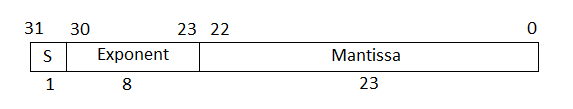Main Content

## Getting Started with HDL Coder Native Floating-Point Support

Native floating-point support in HDL Coder™ enables you to generate code from your floating-point design. If your design has complex math and trigonometric operations or has data with a large dynamic range, use native floating-point.

### Key Features

In your Simulink® model:

• You can have half-precision, single-precision, and double-precision floating-point data types and operations.

• You can have a combination of integer, fixed-point, and floating-point operations. By using Data Type Conversion blocks, you can perform conversions between floating-point and fixed-point data types.

The generated code:

• Complies with the IEEE-754 standard of floating-point arithmetic.

• Is target-independent. You can deploy the code on any generic FPGA or an ASIC.

• Does not require floating-point processing units or hard floating-point DSP blocks on the target ASIC or FPGA.

HDL Coder supports:

• Math and trigonometric functions

• Large subset of Simulink blocks

• Denormal numbers

• Customizing the latency of the floating-point operator

### Numeric Considerations and IEEE-754 Standard Compliance

Native floating point technology in HDL Coder adheres to IEEE standard of floating-point arithmetic. For basic arithmetic operations such as addition, subtraction, multiplication, division, and reciprocal, when you generate HDL code in native floating-point mode, the numeric results obtained match the original Simulink model.

Certain advanced math operations such as exponential, logarithm, and trigonometric operators have machine-specific implementation behaviors because these operators use recurring Taylor series and Remez expression based implementations. When you use these operators in native floating-point mode, the generated HDL code can have relatively small numeric differences from the Simulink model. These numeric differences are within a tolerance range and therefore indicate compliance with the IEEE-754 standard.

To generate code that complies with the IEEE-754 standard, HDL Coder supports:

• Round to nearest rounding mode

• Denormal numbers

• Exceptions such as NaN (Not a Number), Inf, and Zero

• Customization of ULP (Units in the Last Place) and relative accuracy

For more information, see Numeric Considerations with Native Floating-Point.

### Floating Point Types

#### Single Precision

In the IEEE 754–2008 standard, the single-precision floating-point number is 32-bits. The 32-bit number encodes a 1-bit sign, an 8-bit exponent, and a 23-bit mantissa.This graph is the normalized representation for floating-point numbers. You can compute the actual value of a normal number as:

`$value={\left(-1\right)}^{sign}*\left(1+\underset{i=1}{\stackrel{23}{\Sigma }}{b}_{23-i}{2}^{-i}\right)*{2}^{\left(e-127\right)}$`

The exponent field represents the exponent plus a bias of 127. The size of the mantissa is 24 bits. The leading bit is a 1, so the representation encodes the lower 23 bits.

Use single-precision types for applications that require larger dynamic range than half-precision types. Single-precision operations consume less memory and has lower latency than double-precision types.

#### Double Precision

In the IEEE 754–2008 standard, the single-precision floating-point number is 64-bits. The 64-bit number encodes a 1-bit sign, an 11-bit exponent, and a 52-bit mantissa.The exponent field represents the exponent plus a bias of 1023. The size of the mantissa is 53 bits. The leading bit is a 1, so the representation encodes the lower 52 bits.

Use double-precision types for applications that require larger dynamic range, accuracy, and precision. These operations consume larger area on the FPGA and lower target frequency.

#### Half Precision

In the IEEE 754–2008 standard, the half-precision floating-point number is 16-bits. The 16-bit number encodes a 1-bit sign, a 5-bit exponent, and a 10-bit mantissa.The exponent field represents the exponent plus a bias of 15. The size of the mantissa is 11 bits. The leading bit is a 1, so the representation encodes the lower 10 bits.

Use half-precision types for applications that require smaller dynamic range, consumes much less memory, has lower latency, and saves FPGA resources.

When using `half` types, you might want to explicitly set the Output data type of the blocks to `half` instead of the default setting `Inherit: Inherit via internal rule`. To learn how to change the parameters programmatically, see Set HDL Block Parameters for Multiple Blocks Programmatically.

### Data Type Considerations

With native floating-point support, HDL Coder supports code generation from Simulink models that contain floating-point signals and fixed-point signals. You might want to model your design with floating-point types to:

• Implement algorithms that have a large or unknown dynamic range that can fall outside the range of representable fixed-point types.

• Implement complex math and trigonometric operations that are difficult to design in fixed point.

• Obtain a higher precision and better accuracy.

Floating-point designs can potentially occupy more area on the target hardware. In your Simulink model, it is recommended to use floating-point data types in the algorithm data path and fixed-point data types in the algorithm control logic. This figure shows a section of a Simulink model that uses `Single` and fixed-point types. By using Data Type Conversion blocks, you can perform conversions between the single and fixed-point types.## Support

#### Deploying 5G NR Wireless Communications on FPGAs: A Complete MATLAB and Simulink Workflow

Download white paper